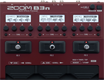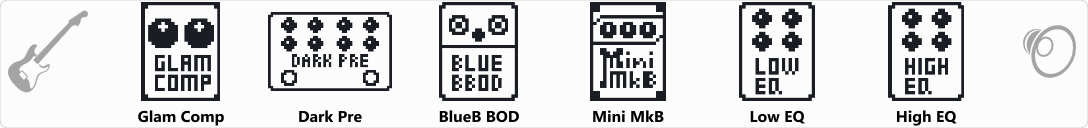# Johno Dunn OD

Discussion in 'Zoom B3n' started by hooham482, May 13, 2021.

1. Johno Dunn ODDevice: Zoom B3n
Firmware: 2.00

Name on device: JDBassOD
Optimized for: Guitar Amp

Effects chain:Effect: "Glam Comp" (Dynamics), active - "yes"
"Comp" = 86
"Shape" = 1
"Volume" = 14
"DryMx" = 61

Effect: "Dark Pre" (Overdrive / Distortion), active - "yes"
"Bass" = 77
"Lo-Mid" = 70
"Hi-Mid" = 83
"Treble" = 17
"Blend" = 50
"Gain" = 50
"Volume" = 65
"Boost" = LO+HI

Effect: "BlueB BOD" (Overdrive / Distortion), active - "yes"
"Gain" = 36
"Nature" = 35
"Blend" = 43
"Volume" = 23

Effect: "Mini MkB" (Amp simulator), active - "yes"
"Gain" = 62
"VNTG" = 52
"Shape" = 0
"Volume" = 43

Effect: "Low EQ" (Filter), active - "yes"
"Type" = HPF
"Frequency" = 20
"Gain" = 10.0
"Volume" = 85

Effect: "High EQ" (Filter), active - "yes"
"Type" = HPF
"Frequency" = 700
"Gain" = 10.0
"Volume" = 85

Patch Volume: 100